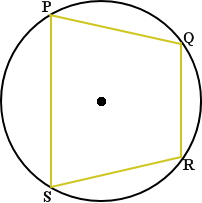Parts of a Circle

Parts of a Circle

A circle is a set of all points in a plane that are all an equal distance from a single point, the center. The distance from a circle's center to a point on the circle is called the radius of the circle. A radius is a line segment with one endpoint at the center of the circle and the other endpoint on the circle. For the circle below AD, DB, and DC are radii of a circle with center D.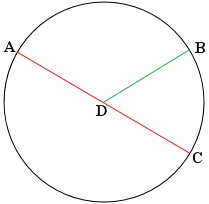A line segment that crosses the circle by passing through the center of the circle is called the diameter. The diameter twice the length of the radius. In the circle above AC is the diameter of the circle.

chord is a segment that also has endpoints on the circle, but the line does not need to cross through the center. On the circle below BC and AC are chords. A diameter is a chord that passes through the center of the circle.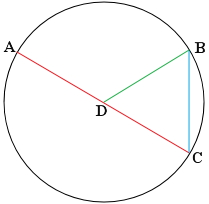secant is a line that intersects with a circle at 2 different points. In the circle below, line E is a secant. A tangent is a line that intersects with the circle at one point. In the circle below line F is a tangent.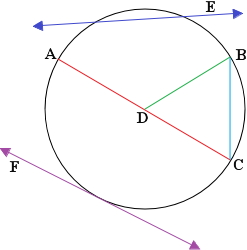Tangents

If a line is tangent to a circle, then it is perpendicular to the radius drawn to the point of tangency.

Example

Jason is located at a point P, 28 feet from the edge of a circular garden. The distance from Jason to a point of tangency on the garden is 56 feet. What is the radius of the garden?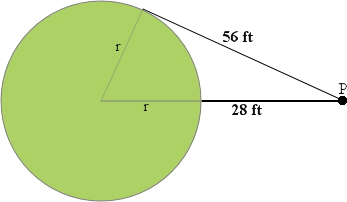(r + 28)= r+ 56r+ 56r + 784 = r+ 3136

r+ 56r - r= 3136 — 784

56r = 2352

r = 42

Arcs

If the measure of angle ADB is less than 180°, then the interior of angle ADB forms the minor arc while the exterior forms the major arc. The measure of an arc is defined by the measure of its central angle. In the example below, the measure of arc BC is 35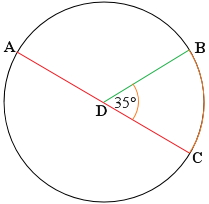Chords of Circles

In the same circle, two minor arcs are congruent if and only if their corresponding chords are congruent, therefore a point D is called the midpoint and arc PQ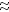arc PR if and only if line PQline PR.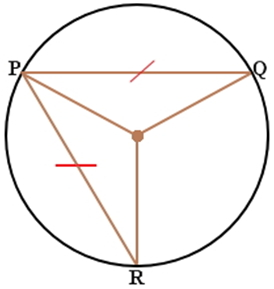If the diameter of a circle is perpendicular to a chord, then the diameter bisects the chord and its arc, therefore XZZY when arc XWarc WY. If one chord is a perpendicular bisector of another chord, then the first chord is a diameter.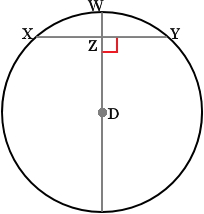Inscribed Angle

If an angle is inscribed in a circle, then its measure is half the measure of its intercepted arc. In the circle below angle QRS =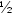of the measure of arc QS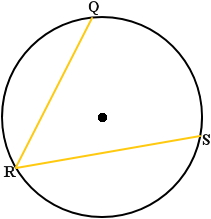If a right triangle is inscribed in a circle, then the hypotenuse may be the diameter of the circle. Conversely, if one side of an inscribed triangle is a diameter of the circle, then the triangle is a right triangle and the angle opposite the diameter is the right angle. In the circle below angle Y is a right angle if and only if line XZ is the diameter of the circle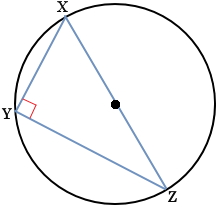A quadrilateral can be inscribed in a circle if and only if its opposite angles are supplementary. In the circle below P, Q, R, and S lie on the circle, with a center at D, if and only if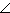P +R = 180andQ +S = 180.